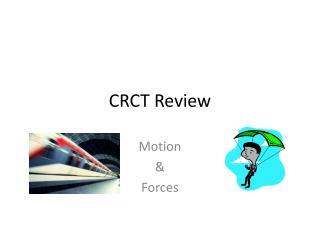DownloadDownload PresentationCRCT Review

# CRCT Review

Download Presentation## CRCT Review

- - - - - - - - - - - - - - - - - - - - - - - - - - - E N D - - - - - - - - - - - - - - - - - - - - - - - - - - -
##### Presentation Transcript

1. CRCT Review Motion & Forces

2. What happens to the motion of an object when the forces acting on it are balanced? • A) The motion changes direction. • B) The motion does not change. • C) The motion speeds up. • D) The motion slows down.

3. What happens to an object if the forces acting on it are unbalanced? • A) Its motion changes. • B) The net force is zero. • C) Its motion does not change. • D) The object always comes to a stop.

4. How is an object’s mass related to the force needed to accelerate the object? • A) mass is not related to the force needed. • B) The smaller the mass, the greater the force needed. • C) The greater the mass, the greater the force needed. • D) The greater the mass, the smaller the force needed.

5. A student pushes four different balls with a pool cue stick. Which ball has the GREATEST inertia? • A) 4 gram ball • B) 15 gram ball • C) 2 gram ball • D) 10 gram ball

6. A person produces the LEAST friction moving across which of these surfaces? • A) carpet • B) ice • C) sand • D) wood floor

7. Air resistance is an example of ____. • A) gravity • B) a noncontact force • C) a net force • D) friction

8. Your mass increases as you grow. How does this affect the gravitational attraction between you and other objects? • A) The force increases. • B) The force decreases. • C) The force stays the same. • D) The force does not change without change in distance.

9. Which two factors affect the gravitational attraction between two objects? • A) density and friction • B) density and distance • C) mass and friction • D) mass and distance

10. What does a simple machine do? • A) It makes work easier. • B) It increases the amount of work done. • C) It decreases the amount of work done. • D) It increases both the force and distance involved in work.

11. Which of the following simple machines is a wedge? • A) wheelbarrow • B) doorknob • C) ax • D) truck ramp

12. Which of the following CANNOT overcome an object’s inertia? A) an unbalanced force B) a zero net force (balanced force) C) gravity D) friction

13. Two objects move closer together, what happens to the gravitational attraction between them? • A) it increases • B) it decreases • C) it doesn’t change • D) it becomes zero

14. Which of the following is a simple machine? • A) bicycle • B) jar lid • C) scissors • D) can opener

15. Which of Newton’s Laws explains the bumper car ride at an amusement park. • a.Newton’s 1st Law • b.Newton’s 2nd Law • c.Newton’s 3rd Law • d.Newton’s Law of Gravitation

16. How do you know an object has moved? • A) it flies • B) it rolls • C) it changes position • D) it can be measured

17. What is the average speed of an object that travels 100 meters in 20 seconds? • A) 4 m/s • B) 8 m/s • C) 5 m/s • D) 10 m/s

18. Which of the following is a unit of speed? • A) h • B) km • C) m/s • D) m/s2

19. A runner takes 1.5 hours to complete a 6 km route. What is the jogger’s average speed? • A) 2.5 km/hr • B) 4.0 km/hr • C) 7.5 km/hr • D) 9.0 km/hr

20. Which of the following is NOT needed to determine the velocity of a moving truck? • A) The mass of the truck. • B) The distance it travels. • C) The time it takes to travel that distance. • D)The direction in which the truck is traveling.